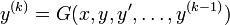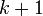Explicit differential equation

An explicit differential equation is a differential equation where the highest-order derivative is explicitly written as a function of the independent variable, dependent variable, and lower order derivatives. Explicitly, if the independent variable is$x$, the dependent variable is$y$, and the order is$k$, it has the form:$y^{(k)} = G(x,y,y',\dots,y^{(k-1)})$
where$G$ is a function of$k +1$ variables.